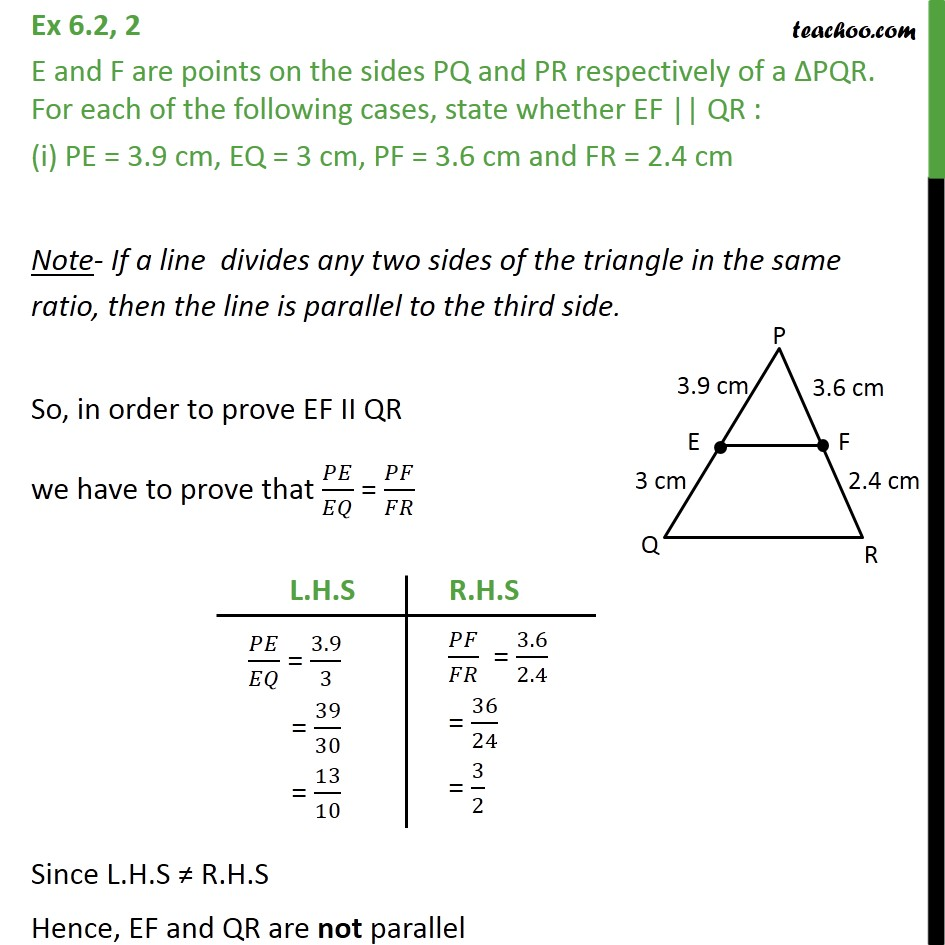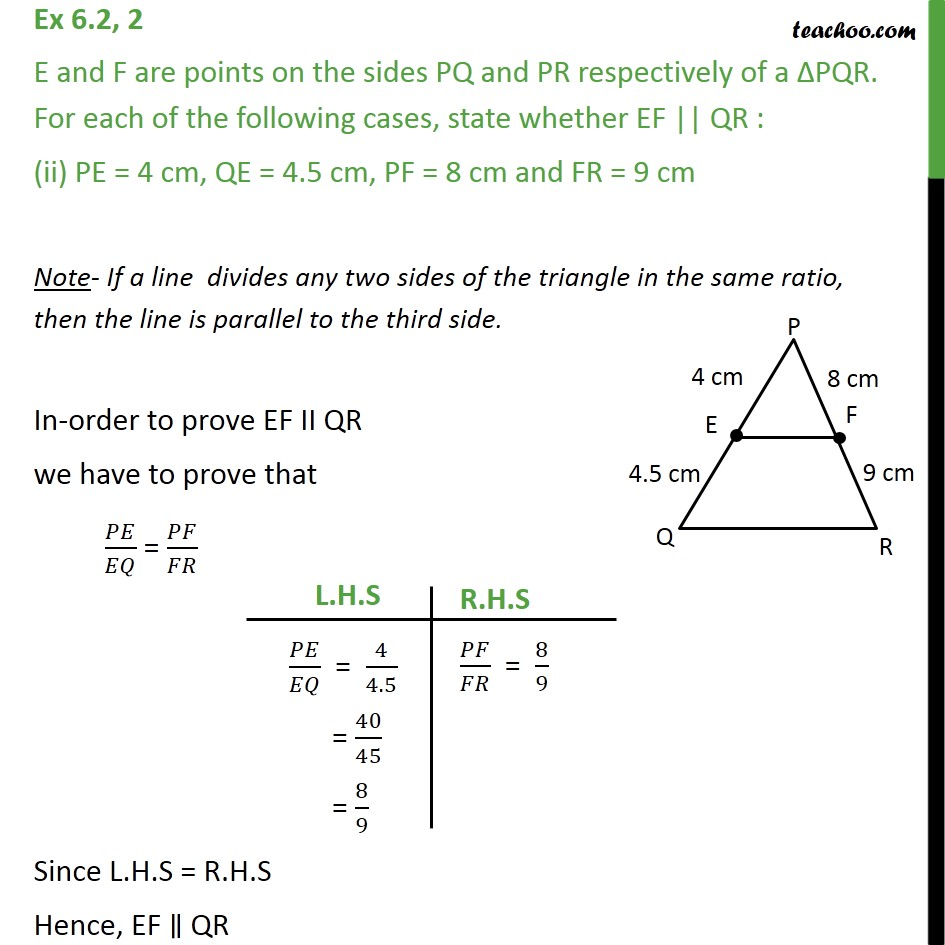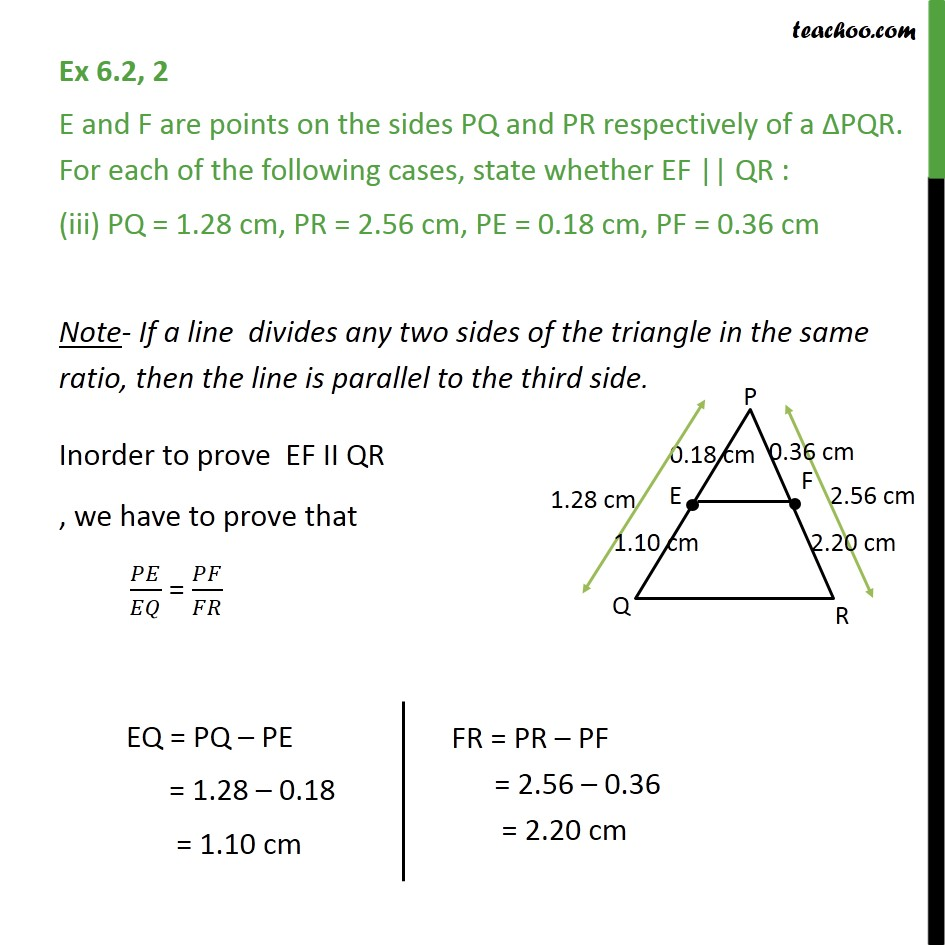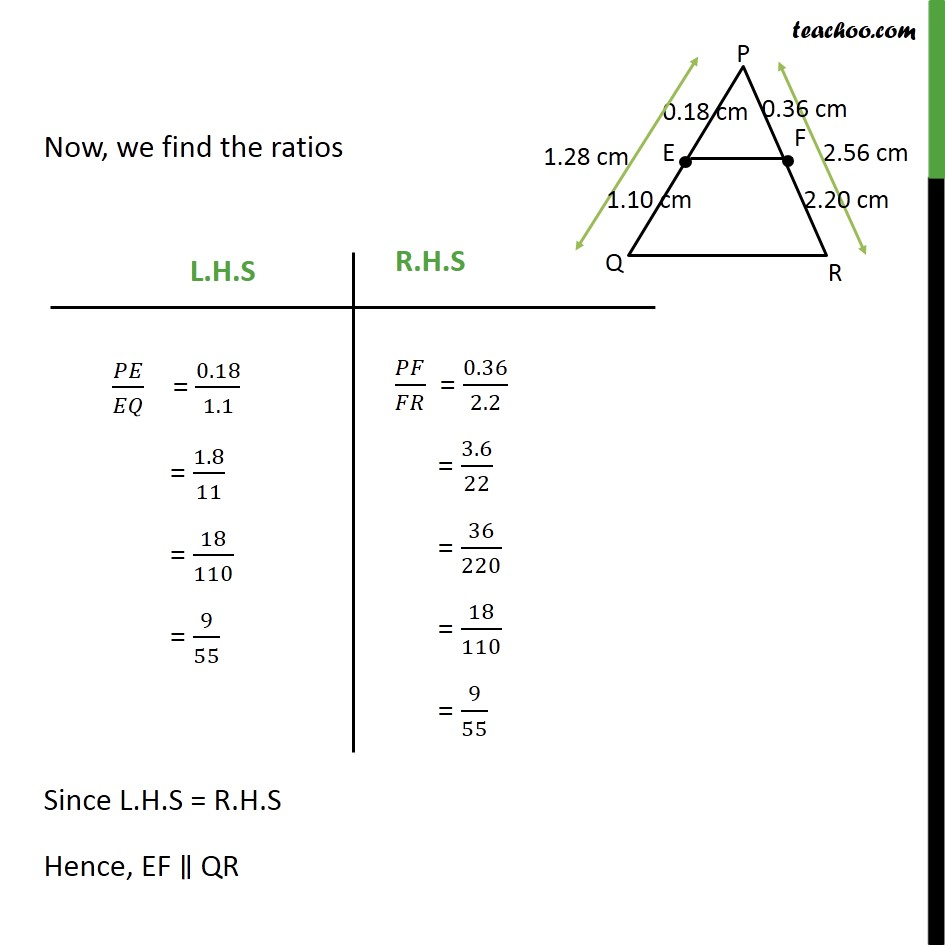Subscribe to our Youtube Channel - https://you.tube/teachoo

1. Chapter 6 Class 10 Triangles
2. Serial order wise
3. Ex 6.2

Transcript

Ex 6.2, 2 E and F are points on the sides PQ and PR respectively of a PQR. For each of the following cases, state whether EF || QR : (i) PE = 3.9 cm, EQ = 3 cm, PF = 3.6 cm and FR = 2.4 cm Note- If a line divides any two sides of the triangle in the same ratio, then the line is parallel to the third side. So, in order to prove EF II QR we have to prove that / = / Since L.H.S R.H.S Hence, EF and QR are not parallel Ex 6.2, 2 E and F are points on the sides PQ and PR respectively of a PQR. For each of the following cases, state whether EF || QR : (ii) PE = 4 cm, QE = 4.5 cm, PF = 8 cm and FR = 9 cm Note- If a line divides any two sides of the triangle in the same ratio, then the line is parallel to the third side. In-order to prove EF II QR we have to prove that / = / Since L.H.S = R.H.S Hence, EF QR Ex 6.2, 2 E and F are points on the sides PQ and PR respectively of a PQR. For each of the following cases, state whether EF || QR : (iii) PQ = 1.28 cm, PR = 2.56 cm, PE = 0.18 cm, PF = 0.36 cm Note- If a line divides any two sides of the triangle in the same ratio, then the line is parallel to the third side. Inorder to prove EF II QR , we have to prove that / = / Now, we find the ratios Since L.H.S = R.H.S Hence, EF QR

Ex 6.2

Chapter 6 Class 10 Triangles
Serial order wise